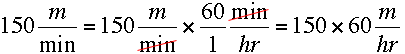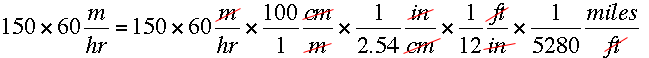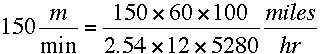SEARCH HOMEMath Central Quandaries & QueriesQuestion from Angela, a parent: If a person is traveling 150 meters per minute, what is their speed in miles per hour?We have two responses for you

Hi Angela,

The units tell me how to proceed. For the time units I know there are 60 minutes in an hour soTo convert metres to miles you need to know a conversion between the distance units in the Imperial system and the metric system. This usually takes me more steps than other people because the only distance conversion between the systems that I remember us that there are 2.54 centimeters in an inch. Also there are 100 centimeters in a metre, 12 inches in a foot and 5280 feet in a mile. ThusHenceHarley

Angela,

Multiply the 150 by the number of minutes in an hour and divide by the number of meters in a mile. Or just go to Google and type 150 meters per minute in miles per hour and the Google server will convert it for you.

Cheers,
Stephen La Rocque.Math Central is supported by the University of Regina and The Pacific Institute for the Mathematical Sciences.